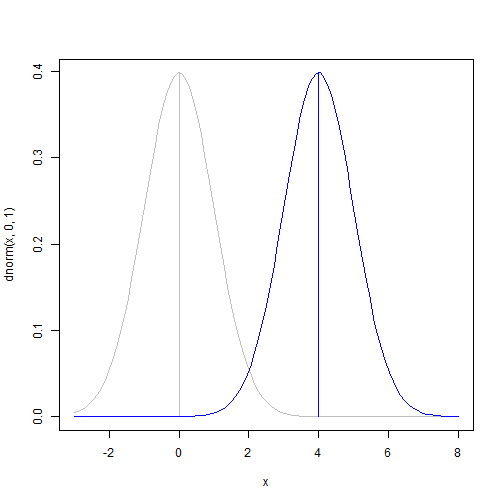# Power, Effect Sizes, and Minimum Detectable Effects

When designing an experiment, we generally want to be able to create an experiment that adequately tests our hypothesis. Accomplishing this requires having sufficient “power” to detect any effects. Power is sometimes also called “sensitivity.” Power refers to the ability of a test (i.e., an analysis of an experiment) to detect a “true effect” that is different from the null hypothesis (e.g., the ability to detect a difference between treatment and control when that difference actually exists).

## Factors influencing power

There are four factors that influence power: sample size, the true effect size, the variance of the effect, and the alpha-threshold (level of significance). The most important factor in power is sample size. Larger samples have more power than small samples, but the gain is power is non-linear. There is a declining marginal return (in terms of power) for each additional unit in the experiment. So designing an experiment trades off power with cost-like considerations. The alpha level (level of significance) also influences power. If we have a more liberal threshold (i.e., a higher alpha level), we have more power to detect the effect. But this higher power is due to the fact that the more liberal treshold also increases our “false positive” rate, where the analysis is more likely to say there is an effect when in fact there is not. So, again, there is a trade-off between detecting a true effect and avoiding false detections.

## Power of a t-test

One of the simplest examples of power involves looking at a common statistical test for analyzing experiments: the t-test. The t-test looks at the difference in means for two groups or the difference between one group and a null hypothesis value (often zero).

t.test()

## Error: argument "x" is missing, with no default

power.t.test()

## Error: exactly one of 'n', 'delta', 'sd', 'power', and 'sig.level' must be
## NULL


## Minimum detectable effect size

Education researcher Howard Bloom has suggested that power is a difficult concept to grasp. He instead suggests we rely on a measure of “minimum detectable effect” (MDE) to discuss experiments. He's probably right. MDE tell us what is the smallest true effect, in standard deviations of the outcome, that is detectable for a given level of power and statistical significance. Because the standard deviation is influenced by sample size, MDE incorporates all of the information of a power calculation but does so in a way that applies to all experiments. That is to say, as long as we can guess at the variance of our outcome, the same sample size considerations apply every time we conduct any experiment.

For a one-tailed test:

sigma <- 1
sigma * qnorm((1 - 0.05)) + sigma * qnorm(0.8)

##  2.486


For a two-tailed test:

sigma * qnorm((1 - (0.5 * 0.05))) + sigma * qnorm(0.8)

##  2.802


We can envision the MDE as the threshold where, for power = .8, 80% of the sampling distribution of the observed effect would be larger than observed effect:

curve(dnorm(x, 0, 1), col = "gray", xlim = c(-3, 8))  # null hypothesis
segments(0, 0, 0, dnorm(0, 0, 1), col = "gray")  # mean
curve(dnorm(x, 4, 1), col = "blue", add = TRUE)  # alternative hypothesis
segments(4, 0, 4, dnorm(4, 4, 1), col = "blue")  # mean# calculate power for a one-tailed test and plot:

p <- qnorm((1 - 0.05), 0, 1) + qnorm(0.8, 0, 1)
segments(p, 0, p, dnorm(p, 4, 1), lwd = 2)

## Error: plot.new has not been called yet


# note how the MDE is larger than the smallest effect that would be considered “significant”:

e <- qnorm((1 - 0.05), 0, 1)
segments(e, 0, e, dnorm(e), lwd = 2)

## Error: plot.new has not been called yet


As in standard power calculations, we still need to calculate the standard deviation of the outcome.

FORTHCOMING